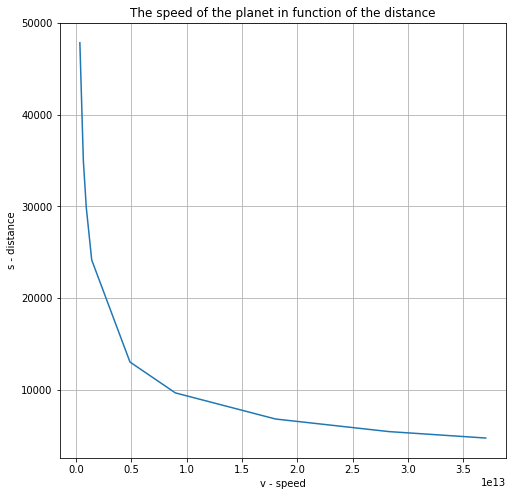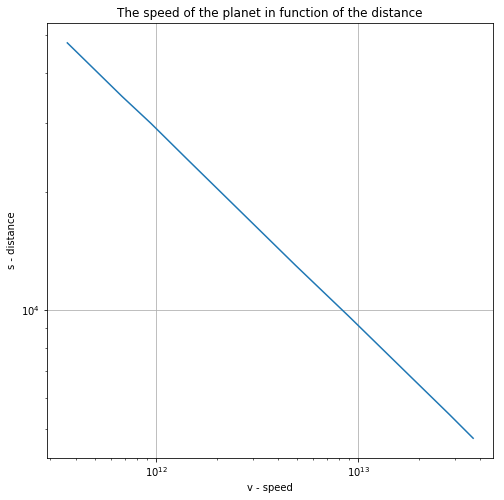Ch-2 Q025 - Physics For Engineers And Scientists - SolutionsON7AMI Amateur Radio, Meteology and Astronomy website. JO10UX# Question 25 - Problems - Chapter 2¶

## Problem:¶

The table printed inside the book cover gives the radii of the orbits of the planers (mean distance from the Sun) and the times required for moving around the orbit (period of revolution).

## Question:¶

a) Calculate the speed of motion of each of the nine planets in its orbit around the Sun. Assume that the orbits are circular.

b) In a logarithmic graph of speed vs. radius, plot the logarithm of the speed of each planet and the logarithm of its radial distance from the Sun as a point. Draw a curve through the nine points. Can you represent this curve by a simple equation?

## Solution:¶

The table:

In :
import numpy as np
import pandas as pd
import matplotlib.pyplot as plt
PlanetName = ['Mercury','Venus', 'Earth', 'Mars', 'Jupiter', 'Saturnus', 'Uranus', 'Neptunus', 'Pluto']
DistanceSun = [57.9e6, 108.0e6, 150.0e6, 228.0e6, 778.0e6, 1430.0e6, 2870.0e6, 4500.0e6, 5890.0e6]
OrbitalPeriod = [0.241, 0.615, 1, 1.88, 11.9, 29.5, 84, 165, 248]
Mass = [3.3e23, 4.87e24, 5.98e24, 6.42e23, 1.9e27, 5.67e26, 8.7e25, 1.03e26, 1.5e22]
Radius = [2439, 6052, 6378, 3393, 71399, 60000, 25400, 24300, 1500]
Gravity = [0.38, 0.91, 1, 0.38, 2.53, 1.07, 0.92, 1.19, 0.045]
RotationPeriod = [58.6, 243, 0.997, 1.026, 0.41, 0.43, 0.65, 0.77, 6.39]
df = pd.DataFrame(data = PlanetDataSet, columns=['PlanetName','DistanceSun','OrbitalPeriod','Mass','Radius','Gravity','RotationPeriod'])
df.set_index('PlanetName', inplace=True)
df

Out:
DistanceSun OrbitalPeriod Mass Radius Gravity RotationPeriod
PlanetName
Mercury 5.790000e+07 0.241 3.300000e+23 2439 0.380 58.600
Venus 1.080000e+08 0.615 4.870000e+24 6052 0.910 243.000
Earth 1.500000e+08 1.000 5.980000e+24 6378 1.000 0.997
Mars 2.280000e+08 1.880 6.420000e+23 3393 0.380 1.026
Jupiter 7.780000e+08 11.900 1.900000e+27 71399 2.530 0.410
Saturnus 1.430000e+09 29.500 5.670000e+26 60000 1.070 0.430
Uranus 2.870000e+09 84.000 8.700000e+25 25400 0.920 0.650
Neptunus 4.500000e+09 165.000 1.030000e+26 24300 1.190 0.770
Pluto 5.890000e+09 248.000 1.500000e+22 1500 0.045 6.390

The length of the orbid is the circumference with the given Distance from the sun.

$$s_{orbit}=2.\pi.r^2$$

The speed of each planet is: $$v_{planet}=\frac{s_{orbit}}{Orbital Period_{(in years)}}$$

So we first add two colums to the table and than fill in the calculated values

In :
# Add two columns
df['Circumference'] = 0
df['Speed(m/s)'] = 0

#iterate the dataset and calculate volume and density
for index, row in df.iterrows():
Circ = 2 * np.pi * (row["DistanceSun"]*1000)
df.loc[index,['Circumference']] = Circ            # add values to the table
Speed = (Circ) / (row['OrbitalPeriod'] * 365.24 * 24 * 3600) # in m/s
df.loc[index,['Speed(m/s)']]  = Speed
print(f'For planet {index} the speed is {Speed:.2e} m/s')

df.sort_values(by=['Speed(m/s)'], ascending = False)

For planet Mercury the speed is 4.78e+04 m/s
For planet Venus the speed is 3.50e+04 m/s
For planet Earth the speed is 2.99e+04 m/s
For planet Mars the speed is 2.41e+04 m/s
For planet Jupiter the speed is 1.30e+04 m/s
For planet Saturnus the speed is 9.65e+03 m/s
For planet Uranus the speed is 6.80e+03 m/s
For planet Neptunus the speed is 5.43e+03 m/s
For planet Pluto the speed is 4.73e+03 m/s

Out:
DistanceSun OrbitalPeriod Mass Radius Gravity RotationPeriod Circumference Speed(m/s)
PlanetName
Mercury 5.790000e+07 0.241 3.300000e+23 2439 0.380 58.600 3.637964e+11 47835.389320
Venus 1.080000e+08 0.615 4.870000e+24 6052 0.910 243.000 6.785840e+11 34965.233391
Earth 1.500000e+08 1.000 5.980000e+24 6378 1.000 0.997 9.424778e+11 29866.136855
Mars 2.280000e+08 1.880 6.420000e+23 3393 0.380 1.026 1.432566e+12 24147.089372
Jupiter 7.780000e+08 11.900 1.900000e+27 71399 2.530 0.410 4.888318e+12 13017.285419
Saturnus 1.430000e+09 29.500 5.670000e+26 60000 1.070 0.430 8.984955e+12 9651.655526
Uranus 2.870000e+09 84.000 8.700000e+25 25400 0.920 0.650 1.803274e+13 6802.842284
Neptunus 4.500000e+09 165.000 1.030000e+26 24300 1.190 0.770 2.827433e+13 5430.206701
Pluto 5.890000e+09 248.000 1.500000e+22 1500 0.045 6.390 3.700796e+13 4728.805002
In :
v = df['Speed(m/s)'].values
s = df['Circumference'].values
plt.figure(figsize=(8,8))
plt.plot(s.tolist(), v.tolist())
plt.grid()
plt.title('The speed of the planet in function of the distance')
plt.xlabel('v - speed')
plt.ylabel('s - distance')
plt.show()This looks like a hyperbolic function.

If we now make the axes logarithmic

In :
plt.figure(figsize=(8,8))
plt.plot(s.tolist(), v.tolist())
plt.grid()
plt.title('The speed of the planet in function of the distance')
plt.xlabel('v - speed')
plt.ylabel('s - distance')
plt.yscale('log')
plt.xscale('log')
plt.show()This confirmed our suspicion that the speed is a logaritmic function of the distance to the sun

The slope of the line is $a = \frac{\bigtriangleup\log(v)}{\bigtriangleup\log(s)}$

The Equation of the line is $y = slope.x + c$

So we first determine the slope by using two arbitrary points

In :
a = (np.log(df.loc['Venus',['Circumference']].values)-np.log(df.loc['Saturnus',['Circumference']].values))
b = (np.log(df.loc['Venus',['Speed(m/s)']].values)-np.log(df.loc['Saturnus',['Speed(m/s)']].values))
slope = b/a
print(f'The slope is: {slope:.2f}')

The slope is: -0.50


So the equation of the line is: $$\log{v}=-0.5\log{s}+\log{c}$$

$$\implies\log{v}= \log{s^{-\frac{1}{2}}}+\log{c}$$

$$\implies \log{v}= \log{c.s^{-\frac{1}{2}}}$$

$$\implies v = c.s^{-\frac{1}{2}}$$

$$\implies v = \frac{c}{\sqrt{r}}$$

 سُوۡرَةُ حٰمٓ السجدة / فُصّلَت ثُمَّ اسْتَوَىٰ إِلَى السَّمَاءِ وَهِيَ دُخَانٌ فَقَالَ لَهَا وَلِلْأَرْضِ ائْتِيَا طَوْعًا أَوْ كَرْهًا قَالَتَا أَتَيْنَا طَائِعِينَ ﴿١١ فَقَضَاهُنَّ سَبْعَ سَمَاوَاتٍ فِي يَوْمَيْنِ وَأَوْحَىٰ فِي كُلِّ سَمَاءٍ أَمْرَهَا ۚ وَزَيَّنَّا السَّمَاءَ الدُّنْيَا بِمَصَابِيحَ وَحِفْظًا ۚ ذَٰلِكَ تَقْدِيرُ الْعَزِيزِ الْعَلِيمِ ﴿١٢ Surah Fussilat: Thereafter turned He to the heaven and it was as smoke, and said Unto it and Unto the earth: come ye twain, willingly or loth. They said: we come willingly. (11) Then He decreed them as seven heavens in two days, and revealed Unto each heaven the command thereof; and We bedecked the nether heaven with lamps and placed therein a guard. That is the ordinance of the Mighty, the Knower. (12) De hemelen en aarde (waren) een samenhangende massa. Wij hebben ze toen van elkaar gescheiden… Wij hebben de hemel tot een beschermend dak gemaakt … en de dag en de nacht, de zon en de maan geschapen. (Koran 41 11:12) Copyright (c) 2000-2020 - Jean Paul Mertens - ON7AMI - formal ON1AMI - Grote Steenweg 86 - 9840 Zevergem - Belgium - Europe